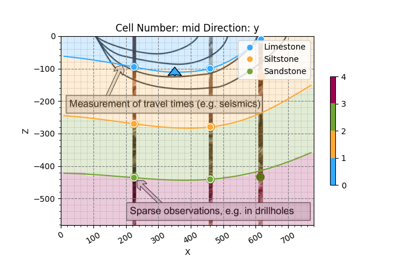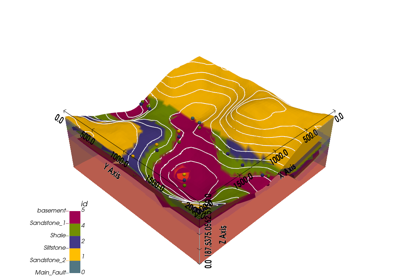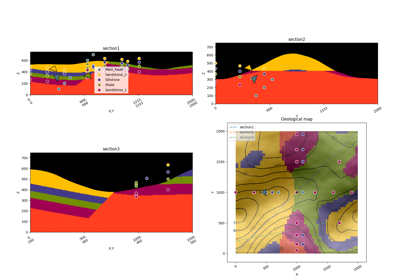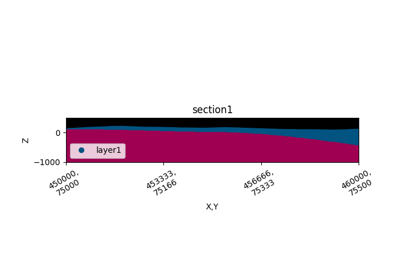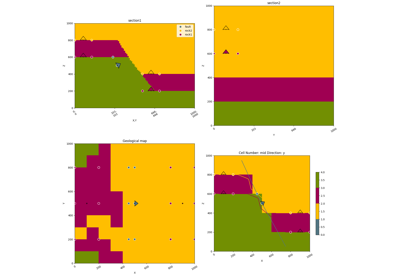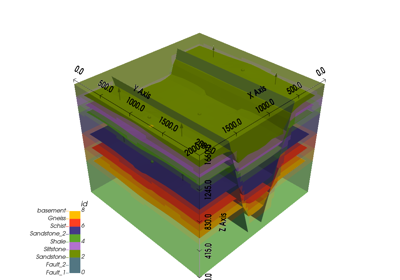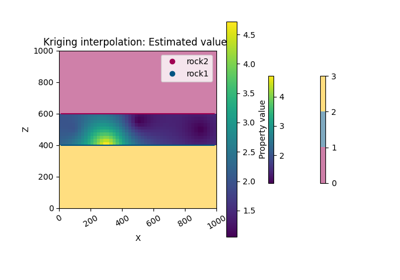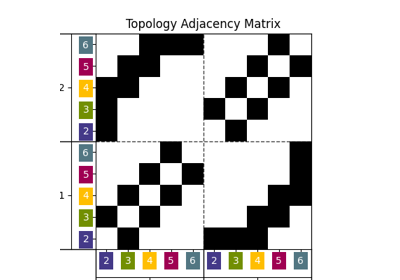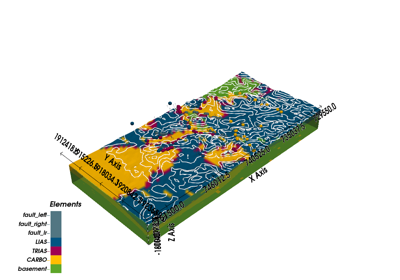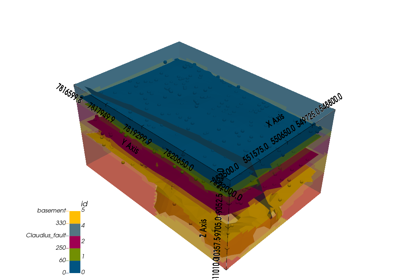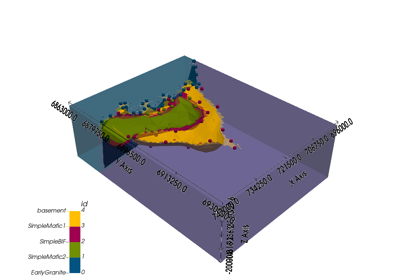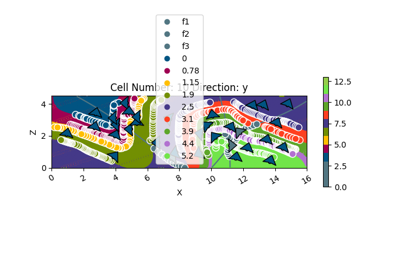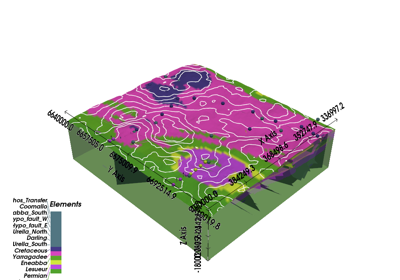# gempy.map_stack_to_surfaces¶

gempy.map_stack_to_surfaces(geo_model: gempy.core.model.Project, mapping_object: Optional[Union[dict, pandas.core.arrays.categorical.Categorical]] = None, set_features=True, sort_geometric_data: bool = True, remove_unused_series=True)[source]

Mapping which surfaces belongs to which geological feature

Parameters
• geo_model (gempy.core.model.Project) – [s0] Container class of all objects that constitute a GemPy model.

• mapping_object (dict, pandas.DataFrame) –

• dict: keys are the series and values the surfaces belonging to that series

• pn.DataFrame: Dataframe with surfaces as index and a column series with the correspondent series name of each surface

• set_features (bool) – If True set missing features from the mapping object

• sort_geometric_data (bool) – If True sort the geometric_data according to the new order of the stack

• remove_unused_series (bool) –

Returns

gempy.core.data.Surfaces

## Examples using gempy.map_stack_to_surfaces¶